# Magnetic force between two parallel current-carrying wires, and the definition of one Ampere

• 1
•
• 2
•
• 3
•
•
•
6
Shares

In the previous article, we have derived an expression for magnetic force on the straight current-carrying conductor placed in a uniform magnetic field. But in this article, we will derive an expression for the magnetic force between the two parallel current-carrying wires. So let’s get started…

## Magnetic force between the two parallel current carrying wires

### When the current flows in same direction

Let’s take two infinitely long straight parallel current carrying wires namely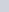and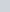, seperated by the distancesuch that the current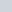andare flowing through them in the same direction, as shown in following figure.

We know that current-carrying wire produces a magnetic field in the form of concentric circles around the wire. So following this statement, first wirewill produce magnetic field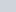and the second wirewill produce magnetic field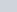. Due to these magnetic fields, each wire will experience magnetic forces on itself. Second wirewill experience magnetic force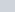due to magnetic fieldof the first wireand first wire will experience magnetic force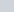due to magnetic fieldof the second wire.

As we know that that the first wire will create a magnetic field, in the shape of circles concentric with the wire. At the place of the second wire, the magnetic fieldis on the page and has a magnitude.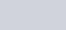Since the second wire carries a current,in upward direction. It will experience a magnetic forcein the presence of the magnetic fieldthat is directed towards the left, see figure above, and it direction can be determined from the right-hand rule. The magnetic forceexerted on a section of lengthon the second wire can be given as-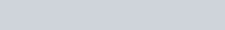Here, we used the fact that the angle between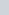and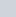is 90°. We also expect from Newton’s Third Law, that an equal and opposite force should be exerted on the first wire as well. As the matter of fact, the second wire will create a magnetic field, that is out of the page at the location of the first wire, whose magnitude can be given as-The magnetic fieldleads to the magnetic forceon the first wire, that points to the right from the right hand rule. On the section of lengthon the first wire, the magnitude of magnetic forcecan be given as-We see thatandboth have equal magnitude. It means, when two parallel straight current-carrying wire has the current in the same direction then they exert equal and opposite attractive forces on each other.

### When the current flows in opposite directions

If the current in the two parallel straight current-carrying wire flows in the opposite direction then there will be no change in the magnitude of the magnetic force that they experienced due to their corresponding magnetic fields. Only the nature of the magnetic force changes. In the case of current in the same direction, the nature of magnetic force is attractive but if the current is in opposite directions, the nature of the magnetic force is repulsive.

## Definition of one Ampere

The attractive force between the two parallel straight current-carrying wires forms the basis for defining the value of one Ampere in their SI unit of an electric current.

Till the year 2019, the one Ampere of an electric current is defined as “the constant current that if maintained in the two parallel straight wires of infinite length, of negligible cross-sectional area, which is placed at one meter apart in vacuum, will produce a magnetic force between this two-wire, equal to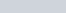per meter of the length.

But recently, the definition of one Ampere has been updated. It is now defined in terms of Coulomb in such a way that the elementary charge has a numerical value of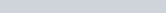and the definition of one Ampere correspond to the coulomb per second.

Watch this video for more visual understanding.

Stay tuned with Laws Of Nature for more useful and interesting content.

/5
5Test your knowledge on "magnetic force on the two parallel current carrying conductors" click start button to begin the quiz

Choose the correct option given below the question and check your score and answers at the end of the quiz.

1 / 5

1. When the current flows in the same direction then the force between the parallel wires is

2 / 5

2. Force per unit length experienced by the two parallel current-carrying wires is given as-

3 / 5

3. When the current flows in opposite directions then the force between the parallel conductors

4 / 5

4. Two parallel current-carrying wires experienced magnetic force due to

5 / 5

5. One ampere of current through each of two parallel conductors of infinite length, separated by one meter in empty space free of other magnetic fields, causes a force of exactly

The average score is 60%

0%